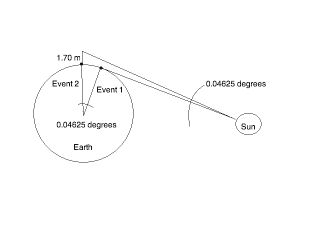# Finding Earth's Radius

## Homework Statement

You are lying down on a beach watching the sunset. You start a stop watch the instant the sun's top disappears below the horizon and stand up, elevating your eyes by 1.70 meters. Exactly 11.1 seconds later, you stop the watch as the sun once again disappears below the horizon. What is the Earth's radius?

## Homework Equations

I think some work with triangles might be involved.

## The Attempt at a Solution

Perhaps the circumference of the Earth could be calculated first. The Earth has rotated $$\frac{11.1 sec}{86400 sec}$$=0.04625 degrees. From here, I'm confused what to do next. I'm pretty sure there's an easier way to do this though.

## Answers and Replies

I did something that I'm not sure is correct. It is in error by about 20% but is on the correct order of magnitude.

Consider what your 0.04625 degrees is telling you. It is the amount of angle the earth has rotated from event 1) sunset on earth surface to event 2) sunset at 1.70 meters above earth surface. So this angle represents a central angle at the earth center. Try to draw that picture, both event 1 and event 2), and see if you can get an answer.

Have you discussed Taylor series expansion of sine and cosine? The angle here is small and I would normally use the first two terms of the Taylor series to get the answer. However, modern calculators probably will give you an answer without roundoff problems which would require this.

Yes, I follow you on this. However, doesn't your proposed solution require that the Earth's circumference be known?

No. What's the definition for the geometric horizon? Draw that line for event 1 and angle zero. Draw the horizon line for event 2 at 1.7 meters height at angle 2.

So we have this then?Sorry, I'm at work (slow day) and your image is being blocked from showing. I'll have to look at it at home in a few hours to see it.

Okay, no worries.

Dick
Science Advisor
Homework Helper
So we have this then?The two lines going to the sun should be the same line. The point 1.7 m above Event 2, Event 1 and the center of the earth are now three vertices of a right triangle.

How do we know the triangle is right?

I'm having trouble getting the picture you're describing, Dick. Can you scribble it out?

Dick
Science Advisor
Homework Helper
Sorry, I'm not very good at scribbling. But there should be a single line to the sun and it should be tangent to the circle at Event 1. That's why it's a right triangle. The tangent is perpendicular to the radius at Event 1. Extend it to the radius at Event 2 and label the distance above Event 2 1.7m. Does that help??

yes, I can see it now.

So, all I need is the distance to the sun, or is that even necessary?

Dick
Science Advisor
Homework Helper
Not necessary. You have a right triangle, you know one leg is R (the earth's radius), the hypotenuse is R+1.7m and you know the included angle. You can solve for R.

Thanks!

That describes what I did to solve it. If you still have trouble visualizing it, try this argument. Pretend your an ant so that your height above horizon is zero. Pretend also that the earth isn't moving. If the sun is at the horizon, then that describes a line tangent to the earth surface and perpendicular to the radial to the earth center. Now move along the earth surface by some amount of central angle (which you calculated). You should be able to convince yourself that in order to be able to see the sun again, you will have to climb a ladder to meet the same horizon line as before. So the horizon line for both angles is the same. Then just solve the triangle problem that Dick points out.

I solved it. The thing that was tripping me up is that I kept drawing the little standing-guy such that he was not parallel to the radius. Solving the following equation for R gave me the solution 5.2 x 106 meters.

cos$$(0.04625 degrees)$$=$$\frac{R meters}{1.70 meters + R meters}$$

Last edited:
Dick
Science Advisor
Homework Helper
I solved it. The thing that was tripping me up is that I kept drawing the little standing-guy such that he was not parallel to the radius. Solving the following equation for R gave me the solution 5.2 x 106 meters.

sin$$(0.04625 degrees)$$=$$\frac{R meters}{1.70 meters + R meters}$$

That's what I get as well. But you meant cos, not sin, right?

Right, LOL.

Geez, and you'd think with a bachelors of science in mathematics, I'd be more careful about transcribing my work!

You'll probably see me around more this summer. I'm starting my masters in the fall, and I want to minor in physics (mathematics will still be my major). I minored in computer science for my undergraduate degree, but I'm not very interested in it anymore.

So I'll be working through some physics courses this summer on my own, hopefully to test out of them.